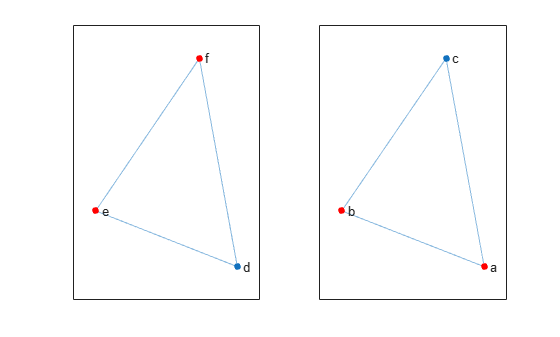# isomorphism

Compute isomorphism between two graphs

## Syntax

``P = isomorphism(G1,G2)``
``P = isomorphism(___,Name,Value)``
``[P,edgeperm] = isomorphism(___)``

## Description

example

````P = isomorphism(G1,G2)` computes a graph isomorphism equivalence relation between graphs `G1` and `G2`, if one exists. If no isomorphism exists, then `P` is an empty array.```

example

````P = isomorphism(___,Name,Value)` specifies additional options with one or more name-value pair arguments. For example, you can specify `'NodeVariables'` and a list of node variables to indicate that the isomorphism must preserve these variables to be valid.```
````[P,edgeperm] = isomorphism(___)` additionally returns a vector of edge permutations, `edgeperm`. This output enables you to preserve edge variables when working with multigraphs.```

## Examples

collapse all

Create and plot two directed graphs, and then calculate the isomorphism relation between them.

```G1 = digraph([1 1 1 2 3 4],[2 3 4 4 4 1]); G2 = digraph([3 3 3 2 1 4],[1 4 2 3 2 2]); subplot(1,2,1) plot(G1) subplot(1,2,2) plot(G2)````p = isomorphism(G1,G2)`
```p = 4×1 3 1 4 2 ```

The result indicates that `reordernodes(G2,p)` has the same structure as `G1`.

Create and plot two graphs, `G1` and `G2`.

```G1 = graph([1 1 1 2 2 3 3 4 5 5 7 7],[2 4 5 3 6 4 7 8 6 8 6 8]); plot(G1,'XData',[1 4 4 1 2 3 3 2],'YData',[4 4 1 1 3 3 2 2])``````G2 = graph({'a' 'a' 'a' 'b' 'b' 'b' 'c' 'c' 'c' 'd' 'd' 'd'}, ... {'g' 'h' 'i' 'g' 'h' 'j' 'g' 'i' 'j' 'h' 'i' 'j'}); plot(G2,'XData',[1 2 2 2 1 2 1 1],'YData',[4 4 3 2 3 1 2 1])```Compute the isomorphism relation between the graphs, if one exists. The result indicates that the graph nodes can be permuted to represent the same graph despite their different labels and layouts.

`p = isomorphism(G1,G2)`
```p = 8×1 1 2 5 3 4 7 6 8 ```

Compute two different isomorphism relations between two graphs. One of the relations preserves a node property, while the other ignores it.

Create two similar graphs. Add a node property `Color` to each of the graphs.

```G1 = graph({'d' 'e' 'f'},{'e' 'f' 'd'}); G1.Nodes.Color = {'blue' 'red' 'red'}'; G2 = graph({'a' 'b' 'c'},{'b' 'c' 'a'}); G2.Nodes.Color = {'red' 'red' 'blue'}';```

Plot the graphs side-by-side in the same figure. Color the nodes red that have `Color = 'red'`.

```subplot(1,2,1) p1 = plot(G1); highlight(p1,{'e' 'f'},'NodeColor','r') subplot(1,2,2) p2 = plot(G2); highlight(p2,{'a' 'b'},'NodeColor','r')```Compute the isomorphism between the graphs, ignoring the `Color` property.

`p = isomorphism(G1,G2)`
```p = 3×1 1 2 3 ```

Compute the isomorphism again, but this time preserve the value of the `Color` property in the comparison. `isomorphism` returns a different permutation that preserves the `Color` property.

`p = isomorphism(G1,G2,'NodeVariables','Color')`
```p = 3×1 3 1 2 ```

View the nodes in `G1` and `G2` that the isomorphism matches together.

`[G1.Nodes.Name, G2.Nodes.Name(p)]`
```ans = 3x2 cell {'d'} {'c'} {'e'} {'a'} {'f'} {'b'} ```

## Input Arguments

collapse all

Input graphs, specified as separate arguments of `graph` or `digraph` objects. Use `graph` to create an undirected graph or `digraph` to create a directed graph.

`G1` and `G2` must be both `graph` objects or both `digraph` objects.

Example: `G1 = graph(1,2)`

Example: `G1 = digraph([1 2],[2 3])`

### Name-Value Arguments

Specify optional comma-separated pairs of `Name,Value` arguments. `Name` is the argument name and `Value` is the corresponding value. `Name` must appear inside quotes. You can specify several name and value pair arguments in any order as `Name1,Value1,...,NameN,ValueN`.

Example: ```P = isomorphism(G1,G2,'NodeVariables',{'Var1' 'Var2'})```

Edge variables to preserve, specified as the comma-separated pair consisting of `'EdgeVariables'` and a character vector, string scalar, cell array of character vectors, or string array. Use this option to specify one or more edge variables that are in both `G1.Edges` and `G2.Edges`. The isomorphism must preserve the specified edge variables in order to be valid.

If `G` is a multigraph, then you can specify the second output `edgeperms` to enable reordering edge variables.

Data Types: `char` | `string` | `cell`

Node variables to preserve, specified as the comma-separated pair consisting of `'NodeVariables'` and a character vector, string scalar, cell array of character vectors, or string array. Use this option to specify one or more node variables that are in both `G1.Nodes` and `G2.Nodes`. The isomorphism must preserve the specified node variables in order to be valid.

Data Types: `char` | `string` | `cell`

## Output Arguments

collapse all

Permutation vector for isomorphism, returned as a column vector when an isomorphism exists or as the empty array `[]` when an isomorphism does not exist. If `P` is not empty, then `reordernodes(G2,P)` has the same structure as `G1`.

Edge permutation, returned as a column vector. When working with multigraphs, the edge permutation vector enables you to preserve edge variables specified by the `'EdgeVariables'` name-value pair. Use these commands to reorder the edge variables of repeated edges:

```[p,edgeperm] = isomorphism(g1,g2,'EdgeVariables',edgevars); g2perm = reordernodes(g2, p); g2perm.Edges(:, 2:end) = g2perm.Edges(edgeperm, 2:end); ```

collapse all

### Graph Isomorphism

Two graphs, `G1` and `G2`, are isomorphic if there exists a permutation of the nodes `P` such that `reordernodes(G2,P)` has the same structure as `G1`.

Two graphs that are isomorphic have similar structure. For example, if a graph contains one cycle, then all graphs isomorphic to that graph also contain one cycle.